# Linear Regression For Impatient Programmer

Back To Index

LinearRegression
In :
import numpy as np
import scipy as scp
import matplotlib.pyplot as plt
import math


In :
datafile = open("LinearData.txt",'r')

In :
data = [ [float(word) for word in line.split()] for line in datafile.readlines()]

In :
data

Out:
[3.36, 15.6495]
In :
n = float(len(data))

In :
print(n)

1000.0

In :
datafile.close()


Next, split the data pairs into lists of x and y

In :
dataX = [XY for XY in data]

In :
dataY = [XY for XY in data]

In :
print(dataX,dataY)

3.36 15.6495


Find average X and Y:

In :
xbar = sum(dataX)/n

In :
ybar = sum(dataY)/n

In :
print("Average X: ",xbar," AverageY: ",ybar)

Average X:  6.651379799999999  AverageY:  38.04975769999991


Now find the standard deviation of X and Y:

In :
varianceX = sum([pow(x-xbar,2) for x in dataX])/(n-1)

In :
stdevX = math.sqrt(varianceX)

In :
print("x variance:", varianceX, "  x standard deviation:", stdevX)

x variance: 17.36767965202397   x standard deviation: 4.167454817034489

In :
varianceY = sum([pow(y-ybar,2) for y in dataY])/(n-1)

In :
stdevY = math.sqrt( varianceY )

In :
print("y variance:", varianceY, "  y standard deviation:", stdevY)

y variance: 758.7477305717524   y standard deviation: 27.545375847349632


And find the covariance and correlation between X and Y

In :
covariance = sum( [(x-xbar)*(y-ybar) for x,y in zip(dataX,dataY)] ) / n

In :
correlation = covariance/(stdevX*stdevY)

In :
print("covariance:", covariance, "  correlation:",correlation)

covariance: 87.22690608192573   correlation: 0.7598552455579058


Next we’ll define a function to make a scatterplot of our data. It’s a good idea to make a plotting function, both for reusability and to contain plot-beautificaiton code.

In :
def splot(dataX,dataY):
fig = plt.figure()
ax = plt.subplot(111)
ax.scatter(dataX, dataY,label='data')
plt.title('Some Very Attractive Title')
plt.xlabel('x')
plt.ylabel('y')
axes = plt.gca()
axes.set_xlim([0,18])
axes.set_ylim([0,190])
ax.legend(loc='upper center', bbox_to_anchor=(0.5, -0.05), shadow=False, ncol=2)
fig.patch.set_facecolor('white') #sets the color of the border
plt.show()

In :
splot(dataX,dataY)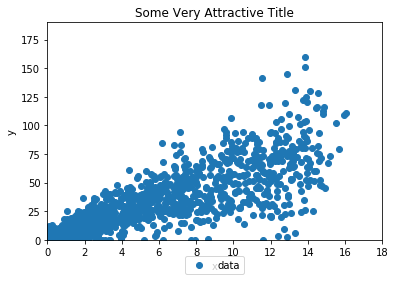Now to make a histogram function. We’ll use this to plot X and Y for now, then other variables.

In :
def hplot(data, nbins, label=''):
fig = plt.figure()
ax = plt.subplot(111)
ax.hist(data,nbins,color='green',alpha=0.8)
plt.title('Histogram')
plt.xlabel(label)
fig.patch.set_facecolor('white') #sets the color of the border
plt.show()

In :
hplot(dataY,10)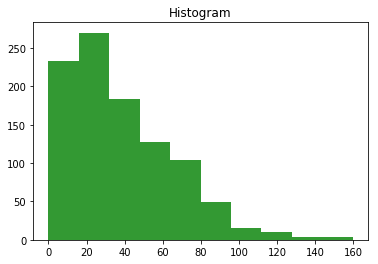Exercise: try changing the number of bins. Also, make a histogram of dataX.

Now we’ll do the least square fit. First we have produce some informaiton.

In :
dataX2 = [x*x for x in dataX]
dataY2 = [y*y for y in dataY]
dataXY = [x*y for x,y in zip(dataX,dataY)]


Now we compute the fit line y = m*x+b, with slope m and vertial intercept b

In :
m = ( sum(dataXY)*n - sum(dataX)*sum(dataY)  )/( sum(dataX2)*n - pow(sum(dataX),2)  )

In :
b = ( sum(dataY)*sum(dataX2) - sum(dataX)*sum(dataXY) )/( sum(dataX2)*n - pow(sum(dataX),2)  )

In :
print("m: ",m,"  b: ",b)

m:  5.027396984032517   b:  4.610630953825091


We would like to plot this line on top of the data. To do that, we first have to make some points that follow the line.

In :
linX = range(20)
linY = [m*x+b for x in linX]


Now make the scatter plot with a line on top

In :
fig = plt.figure()
ax = plt.subplot(111)
ax.scatter(dataX, dataY,label='data')
ax.plot(linX, linY, color='red', label='Fit Line',linewidth=4.0)##New: fit line
plt.title('Least Square fit to the data')
plt.xlabel('x')
plt.ylabel('y')
axes = plt.gca()
axes.set_xlim([0,18])
axes.set_ylim([0,190])
ax.legend(loc='upper center', bbox_to_anchor=(0.5, -0.05), shadow=False, ncol=2)
fig.patch.set_facecolor('white')
plt.show()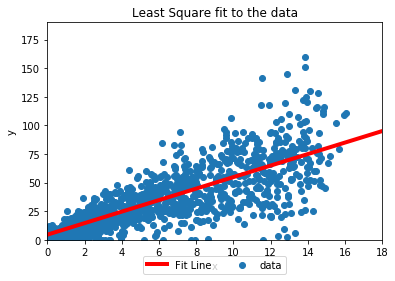First thing to with this fit line is to check how well it explains the data. R^2 gives a measure of the goodness of the fit. It tells us what proportion of the variance in Y is explained by the model. Ideally R^2 would be 1.

In :
SS_residuals = sum( [pow(y - (m*x+b),2) for x,y in zip(dataX,dataY) ]  )
SS_total = sum([pow(y-ybar,2) for y in dataY])
R_squared = 1.0 - (SS_residuals/SS_total)
print(R_squared)

0.5785364886426594


That’s pretty mediocer, which is to be expected in a system as noisy as this one.

We can also select a slice of the plot, or the whole thing, and view the residuals. Again, these are the vertical distances from the fit line.

In :
residuals = [y - m*x+b for x,y in zip(dataX,dataY) if x < 4]
hplot(residuals,15)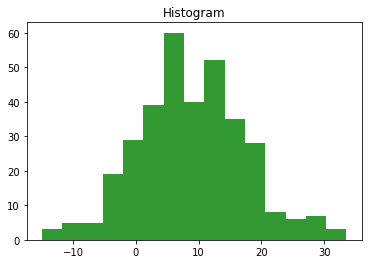We can project the data along the fit line by finding the perpendicular distances from the points and the fit line and then making a histogram of those.

In :
perp_dist = [ (y - (m*x+b))/math.sqrt(1.0+m*m) for x,y in zip(dataX,dataY) ]

In :
hplot(perp_dist,25)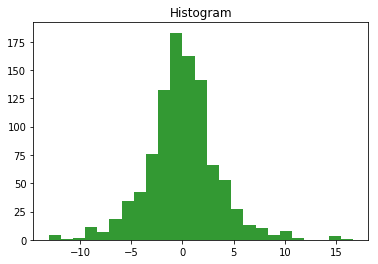This gives us a very narrow peak so we can see the spread around our model line.

In [ ]:



Back To Index